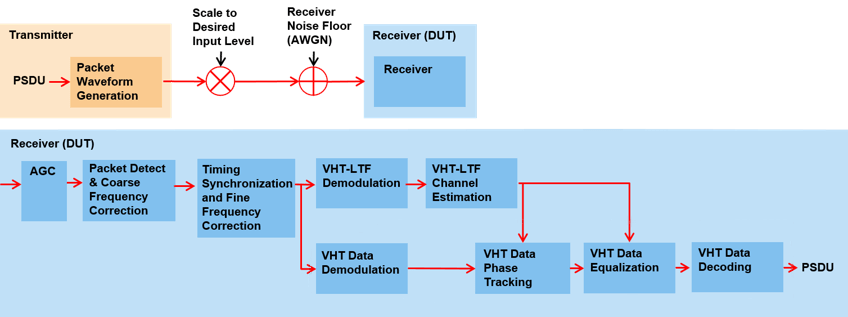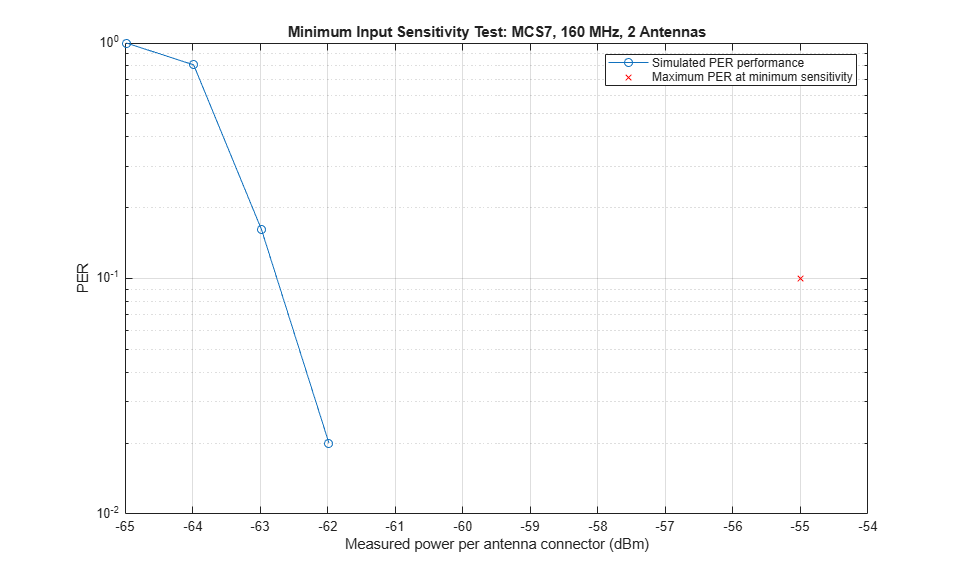# 802.11ac Receiver Minimum Input Sensitivity Test

This example shows how to measure the receiver minimum input sensitivity as specified in Section 21.3.18.1 of the IEEE Std 802.11™-2020 standard [ 1 ].

### Introduction

The receiver minimum sensitivity test ensures a device under test (DUT) receives data with a defined maximum packet error rate (PER) of 10% at a defined minimum signal power. The minimum signal power depends on the channel bandwidth and modulation and coding scheme (MCS) as specified in Table 21-25 of IEEE Std 802.11™-2020 [ 1 ]:When the test is performed with hardware, each input antenna port on the DUT is connected through a cable to a single output antenna port of a transmitter. To perform the test, specify these parameters for the test waveform:

• Number of spatial streams - equal to the number of transmit antennas

• PSDU length, in bytes - 4096

• Space-time block coding (STBC) - disabled

• Guard interval, in nanoseconds - 800

• Channel coding - binary convolutional coding (BCC)

This example shows how to simulate the test by using WLAN Toolbox™. VHT packets stimulate a receiver at a range of input levels below the minimum sensitivity level. The example then measures the packet error rate for each sensitivity level.

The example simulates the test by performing these steps over a range of sensitivity levels:

• Generate and scale packets to the desired signal level

• Add white Gaussian noise is to create a noise floor at the receiver

• Demodulate the noisy packets to recover PSDUs.

• Compare recovered PSDUs to those transmitted to determine the number of packet errors and hence the packet error rate.

Automatic gain control (AGC), packet detection, timing synchronization, carrier frequency offset correction, noise estimation and phase tracking are performed by the example receiver. This diagram demonstrates processing for each packet:### Test Parameters

Configure a transmission for the test by using a VHT configuration object. This example measures the minimum sensitivity for a 160 MHz transmission with 64-QAM rate 5/6 modulation and coding. The simulated DUT has 2 receive antennas. Test different configurations by changing these parameters.

```cfgVHT = wlanVHTConfig; % Create VHT transmission configuration cfgVHT.ChannelBandwidth = 'CBW160'; % Bandwidth cfgVHT.MCS = 7; % 64-QAM, rate 5/6 NumReceiveAntennas = 2; % Number of receive antennas ```

The test requires these fixed transmission parameters.

```cfgVHT.APEPLength = 4096; % Bytes cfgVHT.STBC = false; cfgVHT.NumTransmitAntennas = NumReceiveAntennas; cfgVHT.NumSpaceTimeStreams = NumReceiveAntennas; cfgVHT.SpatialMapping = 'Direct'; cfgVHT.GuardInterval = 'Long'; ```

### Simulation Parameters

A receiver processes VHT packets at a range of input levels below the minimum input sensitivity level. Specify the range of offsets to test in the vector `testInputLevelOffsets`.

```testInputLevelOffsets = [-10 -9 -8 -7]; % dB ```

Control the number of packets tested at each sensitivity by specifying these parameters:

1. `maxNumErrors` is the maximum number of packet errors simulated at each input level. When the number of packet errors reaches this limit, the simulation at this sensitivity level is complete.

2. `maxNumPackets` is the maximum number of packets simulated at each input level and limits the length of the simulation if the packet error limit is not reached.

The numbers chosen in this example lead to a very short simulation. Increase `maxNumErrors` and `maxNumPackets` for meaningful results.

```maxNumErrors = 20; maxNumPackets = 200; ```

### Signal Power Setup

The minimum sensitivity test specifies a maximum PER for a measured input level per receive antenna. In this simulation the receiver processes a test signal with a specified input level in dBm. Generate the test signal using the `wlanWaveformGenerator` function. The `wlanWaveformGenerator` function normalizes the waveform such that the power for all antennas sums to 0 dBm. Therefore, scale the output of the waveform generator to create the desired input level.

```% Receiver minimum input level sensitivity for 20 MHz, Table 21-25. The % sensitivity increases by 3dB for double the bandwidth. rxMinSensitivityTable = [-82 -79 -77 -74 -70 -66 -65 -64 -59 -57]; % dBm % Get minimum input sensitivity given MCS and bandwidth fs = wlanSampleRate(cfgVHT); % Baseband sampling rate (Hz) B = floor(10*log10((fs/20e6))); % Scalar for bandwidth rxMinSensitivity = rxMinSensitivityTable(cfgVHT.MCS+1)+B; % dBm disp(['Minimum sensitivity for MCS' num2str(cfgVHT.MCS) ', ' ... num2str(fs/1e6) ' MHz: ' num2str(rxMinSensitivity,'%2.1f') ' dBm']) ```
```Minimum sensitivity for MCS7, 160 MHz: -55.0 dBm ```

Define the range of input levels below the minimum level to test using `testInputLevels`.

```testInputLevels = rxMinSensitivity+testInputLevelOffsets; % dBm ```

Calculate a voltage scalar, `A`, to scale the generated waveform for each test level. The power per receive antenna port is measured during the simulation to confirm the input signal level is correct.

```A = 10.^((testInputLevels-30)/20); % Voltage gain (attenuation) A = A*sqrt(cfgVHT.NumTransmitAntennas); % Account for generator scaling ```

### Noise Configuration

Add thermal noise at the receiver. The height of the noise floor determines the SNR at the receiver, as the input signal level is fixed for this test. The noise figure of the receiver determines the level of noise floor.

```NF = 6; % Noise figure (dB) T = 290; % Ambient temperature (K) BW = fs; % Bandwidth (Hz) k = 1.3806e-23; % Boltzmann constant (J/K) noiseFloor = 10*log10(k*T*BW)+NF; % dB disp(['Receiver noise floor: ' num2str(noiseFloor+30,'%2.1f') ' dBm']) ```
```Receiver noise floor: -85.9 dBm ```

Add noise to the waveform using an AWGN channel, `comm.AWGNChannel`.

```awgnChannel = comm.AWGNChannel('NoiseMethod','Variance', ... 'Variance',10^(noiseFloor/10)); ```

### Input Level Sensitivity Simulation

Calculate the packet error rate for each input level by simulating multiple packets.

For each packet perform the following processing steps:

1. Create and encode a PSDU to create a single packet waveform.

2. Create the desired input level in dBm by scaling the waveform.

3. Measure the power of the received waveform.

5. Boost the signal prior to processing by passing through an automatic gain control.

6. Detect the packet.

7. Estimate and correct coarse carrier frequency offset.

8. Establish fine timing synchronization.

9. Estimate and correct fine carrier frequency offset.

10. Extract and OFDM demodulate the VHT-LTF and perform channel estimation.

11. Extract the VHT Data field and recover the PSDU.

```ind = wlanFieldIndices(cfgVHT); % For accessing fields within the packet chanBW = cfgVHT.ChannelBandwidth; rng(0); % Set random state for repeatability agc = comm.AGC; % Automatic gain control S = numel(testInputLevels); packetErrorRate = zeros(S,1); rxAntennaPower = zeros(S,1); for i=1:S disp(['Simulating ' num2str(testInputLevels(i),'%2.1f') ... ' dBm input level...']); % Loop to simulate multiple packets numPacketErrors = 0; measuredPower = zeros(maxNumPackets,1); % Average power per antenna numPkt = 1; % Index of packet transmitted while numPacketErrors<=maxNumErrors && numPkt<=maxNumPackets % Generate a packet waveform txPSDU = randi([0 1],cfgVHT.PSDULength*8,1); % PSDULength in bytes tx = wlanWaveformGenerator(txPSDU,cfgVHT); % Scale input signal to desired level rx = tx.*A(i); % Measure the average power at the antenna connector in Watts measuredPower(numPkt) = mean(mean(rx.*conj(rx))); % Add noise floor at receiver rx = awgnChannel(rx); % Pass each channel through AGC for ic = 1:size(rx,2) rx(:,ic) = agc(rx(:,ic)); reset(agc); end % Packet detect and determine coarse packet offset coarsePktOffset = wlanPacketDetect(rx,chanBW); if isempty(coarsePktOffset) % If empty no L-STF detected; packet error numPacketErrors = numPacketErrors+1; numPkt = numPkt+1; continue; % Go to next loop iteration end % Extract L-STF and perform coarse frequency offset correction lstf = rx(coarsePktOffset+(ind.LSTF(1):ind.LSTF(2)),:); coarseFreqOff = wlanCoarseCFOEstimate(lstf,chanBW); rx = frequencyOffset(rx,fs,-coarseFreqOff); % Extract the non-HT fields and determine fine packet offset nonhtfields = rx(coarsePktOffset+(ind.LSTF(1):ind.LSIG(2)),:); finePktOffset = wlanSymbolTimingEstimate(nonhtfields,chanBW); % Determine final packet offset pktOffset = coarsePktOffset+finePktOffset; % if packet detected out of a reasonable range (>50 samples); % packet error if pktOffset>50 numPacketErrors = numPacketErrors+1; numPkt = numPkt+1; continue; % Go to next loop iteration end % Extract L-LTF and perform fine frequency offset correction lltf = rx(pktOffset+(ind.LLTF(1):ind.LLTF(2)),:); fineFreqOff = wlanFineCFOEstimate(lltf,chanBW); rx = frequencyOffset(rx,fs,-fineFreqOff); % Extract VHT-LTF samples from the waveform, demodulate and perform % channel estimation vhtltf = rx(pktOffset+(ind.VHTLTF(1):ind.VHTLTF(2)),:); vhtltfDemod = wlanVHTLTFDemodulate(vhtltf,cfgVHT); chanEst = wlanVHTLTFChannelEstimate(vhtltfDemod,cfgVHT); % Get single stream channel estimate chanEstSSPilots = vhtSingleStreamChannelEstimate(vhtltfDemod,cfgVHT); % Extract VHT Data samples from the waveform vhtdata = rx(pktOffset+(ind.VHTData(1):ind.VHTData(2)),:); % Estimate the noise power in VHT data field nEstVHT = vhtNoiseEstimate(vhtdata,chanEstSSPilots,cfgVHT); % Recover the transmitted PSDU in VHT Data rxPSDU = wlanVHTDataRecover(vhtdata,chanEst,nEstVHT,cfgVHT, ... 'LDPCDecodingMethod','norm-min-sum'); % Determine if any bits are in error, i.e. a packet error packetError = any(biterr(txPSDU,rxPSDU)); numPacketErrors = numPacketErrors+packetError; numPkt = numPkt+1; end % Calculate packet error rate (PER) at input level point packetErrorRate(i) = numPacketErrors/(numPkt-1); disp([' Completed after ' ... num2str(numPkt-1) ' packets, PER: ' ... num2str(packetErrorRate(i))]); % Calculate average input power per antenna rxAntennaPower(i) = 10*log10(mean(measuredPower(1:(numPkt-1))))+30; disp([' Measured antenna connector power: ' ... num2str(rxAntennaPower(i),'%2.1f') ' dBm']); end ```
```Simulating -65.0 dBm input level... Completed after 21 packets, PER: 1 Measured antenna connector power: -65.0 dBm Simulating -64.0 dBm input level... Completed after 26 packets, PER: 0.80769 Measured antenna connector power: -64.0 dBm Simulating -63.0 dBm input level... Completed after 130 packets, PER: 0.16154 Measured antenna connector power: -63.0 dBm Simulating -62.0 dBm input level... Completed after 200 packets, PER: 0.02 Measured antenna connector power: -62.0 dBm ```

### Analysis and Further Exploration

Plot the PER for tested input signal levels with the maximum PER at minimum sensitivity.

```figure semilogy(rxAntennaPower,packetErrorRate,'o-') hold on semilogy(rxMinSensitivity,0.1,'rx') currentxlim = xlim(gca); xlim([currentxlim(1) currentxlim(2)+1]) grid on xlabel('Measured power per antenna connector (dBm)'); ylabel('PER'); legend('Simulated PER performance','Maximum PER at minimum sensitivity'); title(sprintf(['Minimum Input Sensitivity Test: MCS%d, %d MHz, ' ... '%d Antennas'],cfgVHT.MCS,fs/1e6,cfgVHT.NumTransmitAntennas)) ```The plot reveals the simulated 10% PER is just under 8 dB lower than the minimum sensitivity specified by the test. This difference is due to the implementation margin allowed by the test. The implementation margin allows for algorithmic degradations due to impairments and the receiver noise figure when compared to ideal AWGN performance [ 2 ]. In this example only AWGN is added as an impairment. Therefore, only the algorithmic performance of front-end synchronization, channel estimation and phase tracking in the presence of AWGN use the implementation margin. If more impairments are included in the simulation the PER waterfall in the plot will move right towards the minimum sensitivity and the margin will decrease.

The number of packets tested at each SNR point is controlled by two parameters; `maxNumErrors` and `maxNumPackets`. For meaningful results, you should use larger numbers than those used in this example.

### Appendix

This example uses the following helper functions:

### Selected Bibliography

1. IEEE Std 802.11™-2020. IEEE Standard for Information Technology - Telecommunications and Information Exchange between Systems - Local and Metropolitan Area Networks - Specific Requirements - Part 11: Wireless LAN Medium Access Control (MAC) and Physical Layer (PHY) Specifications.

2. Perahia, Eldad, and Robert Stacey. Next Generation Wireless LANS: 802.11n and 802.11ac. Cambridge University Press, 2013.